# Noorul Huda

Timeline created by lmkmath
In History
• 1,800 BCE

# Babylonian MathematicsThe Babylonians, who were famous for their astronomical observations and calculations (aided by their invention of the abacus), used a sexagesimal (base-60) positional numeral system inherited from either the Sumerian or the Eblaite civilizations. The Babylonians solved quadratic equations with the completing the square method.
• -569 BCE

# Pythagoras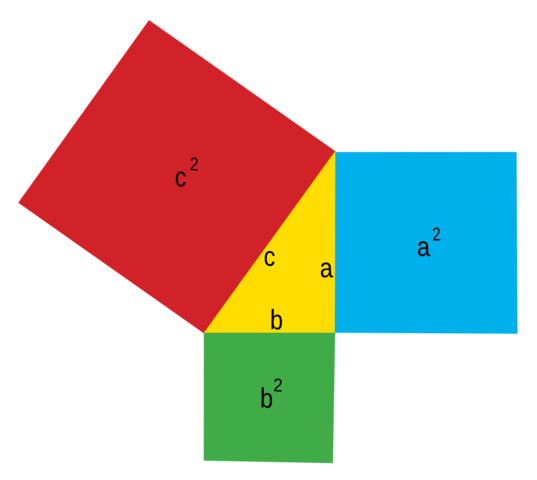Greek mathematician Pythagoras' followers use geometric, visual strategies to solve equations.Pythagoras of Samos was an Ionian Greek philosopher and the eponymous founder of the Pythagoreanism movement.Pythagoras is often referred to as the first pure mathematician. He was born on the island of Samos, Greece in 569 BC. Various writings place his death between 500 BC and 475 BC in Metapontum, Lucania, Italy.
• 288

# Diophantus of Alexandria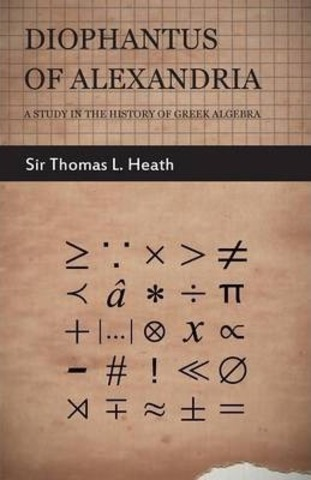Greek mathematician Diophantus of Alexandria begins replacing statements with symbols.Diophantus was a Hellenistic Greek (or possibly Egyptian, Jewish or even Chaldean) mathematician who lived in Alexandria during the 3rd Century CE. He is sometimes called “the father of algebra”, and wrote an influential series of books called the “Arithmetica”, a collection of algebraic problems which greatly influenced the subsequent development of number theory.
• 1539

# Girolamo Cardano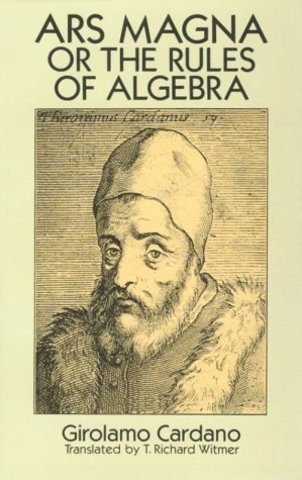Girolamo Cardano writes a book containing solutions to cubic and quadratic equations. In 1539 he published two books on arithmetic embodying his popular lectures, the more important being Practica arithmetica et mensurandi singularis (“Practice of Mathematics and Individual Measurements”). His Ars magna(1545) contained the solution of the cubic equation, for which he was indebted to the Venetian mathematician.
• # Francois VieteFrancois Viete starts uses letters to replace variables and uses the +/- signs to represent addition and subtraction.François Viète, Seigneur de la Bigotière, was a French mathematician whose work on new algebra was an important step towards modern algebra, due to its innovative use of letters as parameters in equations.
• # Isaac Newton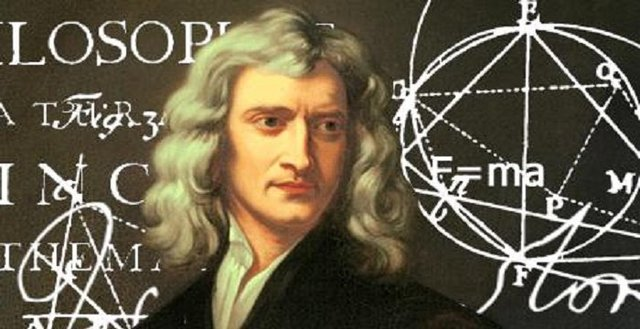Isaac Newton creates the law of universal gravitation.
• # Niels Henrik AbelNorwegian mathematician Niels Henrik Abel proves that there is no general formula that solves all quintic equations. Niels Henrik Abel was a Norwegian mathematician who made pioneering contributions in a variety of fields. His most famous single result is the first complete proof demonstrating the impossibility of solving the general quintic equation in radicals.
• # Muhammad Ibn Musa Al-Khwarizmi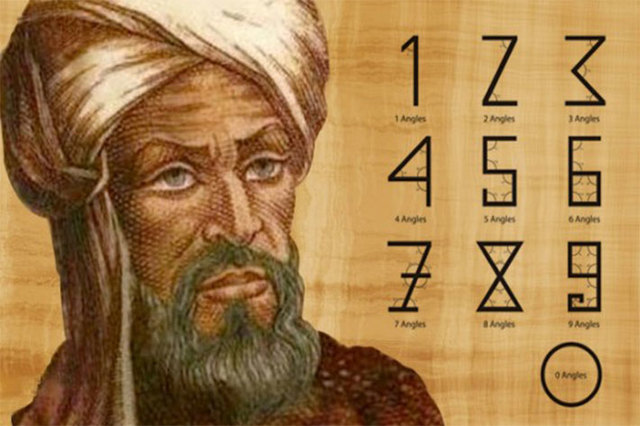In Iraq, Muhammad Ibn Musa Al-Khwarizmi writes a book containing the first clear explanation of solving equations by doing the same operation on both sides.Al-Khwarizmi developed the concept of the algorithm in mathematics.Today, people use algorithms to do addition and long division, principles that are found in Al-Khwarizmi’s text written about 1200 years ago.
• # Albert EinsteinAlbert Einstein published Theory of Relativity.
In 1905, Albert Einstein determined that the laws of physics are the same for all non-accelerating observers, and that the speed of light in a vacuum was independent of the motion of all observers. This was the theory of special relativity.
• # Georges LemaîtreGeorges Lemaître proposes the Big Bang Theory.In 1927, the Belgian Catholic priest Georges Lemaître proposed an expanding model for the universe to explain the observed redshifts of spiral nebulae, and calculated the Hubble law. He based his theory on the work of Einstein and De Sitter, and independently derived Friedmann's equations for an expanding universe.